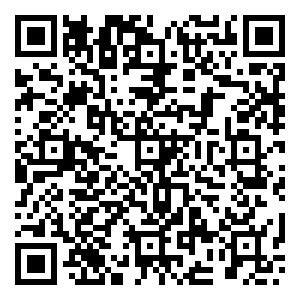## 留言板引用本文: 陈立军, 潘正军, 陈孝如. 无人机GPS欺骗干扰攻击对策[J]. 全球定位系统, 2023, 48(4): 91-98.CHEN Lijun, PAN Zhengjun, CHEN Xiaoru. Countermeasure of UAV GPS spoofing jamming attcck[J]. GNSS World of China, 2023, 48(4): 91-98. doi: 10.12265/j.gnss.2023026
 Citation: CHEN Lijun, PAN Zhengjun, CHEN Xiaoru. Countermeasure of UAV GPS spoofing jamming attcck[J]. GNSS World of China, 2023, 48(4): 91-98.## 无人机GPS欺骗干扰攻击对策

##### doi: 10.12265/j.gnss.2023026

###### 通讯作者: 陈立军 E-mail:372158286@qq.com
• 中图分类号: P228.4

## Countermeasure of UAV GPS spoofing jamming attcck

• 摘要: 自主和半自主的无人机群(unmanned aerial vehicles，UAVs)开始引发大量的研究兴趣和来自各种民用应用领域的需求，然而，为成功执行各种任务，无人机群需要GPS的信号. 民用GPS信号是未加密和未认证的，这为GPS欺骗攻击的实施提供了便利，在这些攻击中，对手通过模仿真实的GPS信号，并将其广播给目标无人机，以改变其航线，迫使其降落或坠毁. 在这项研究中，提出了一种GPS欺骗检测机制，能够检测单发射器和多发射器的GPS欺骗攻击，以防止改变其航线或坠毁. GPS欺骗是基于比较每两个蜂群成员之间从其GPS坐标计算出的距离和同一蜂群成员之间从脉冲无线电超宽带测距获得的距离，如果距离的差异大于选定的阈值，则宣布检测到GPS欺骗攻击. 通过案例证明：检测机制优于现存的检测技术，它无需修改原有的天线和安装额外的硬件，便可检测GPS欺骗攻击，并且也不需要复杂的计算和通信开销，减少了误报，使检测机制更加可靠.

• 图  1  单发射器GPS欺骗攻击

Figure  1.  Single transmitter GPS spoofing attack

图  2  多发射机GPS欺骗攻击

Figure  2.  Multi-transmitter GPS spoofing attack

图  3  UAV到集群中其他UAVs的IR-UWB测距数据采集过程

Figure  3.  IR-UWB ranging data acquisition process from one UAV to other UAVs in the cluster

表  1  GPS欺骗检测机制

Table  1.   GPS spoofing detection mechanism

 机制 方法 限制和缺点 检测率 基于车辆网络(vehicle network，VN) 到达时间相关性 仅适用于VN；需要车对车通信 不适用 基于摄像头的视频流 帧和坐标之间的相关性 速度和地形可靠；要求摄像机始终指向下方 不适用 Crowd-GPS-Sec 到达时间、信号财产和广播式自动相关监视(automatic dependent surveillance - broadcast，ASD-B)信息分析 需要额外的地面基础设施 75.0 % 基于蜂窝网络 位置有效性交叉检查 需要蜂窝模块；需要蜂窝网络覆盖 95.0 % 基于陀螺仪和加速计 位置估计 需要额外的运动传感器进行精确检测 96.0 % 基于IMU 机器学习 计算开销；受初始训练数据集的限制 96.3 % 多接收器 位置比较 需要额外的硬件；不适用于小型无人机 99.0 % 天线阵列 GPS信号的到达方向 需要额外的硬件；不适用于小型无人机 99.0 % 基于蜂窝网络 位置验证 需要额外的蜂窝模块；不适合农村地区 100.0 % 本文的GPS欺骗检测机制 距离验证 不需要额外的硬件，适用于小型、中型无人机 100.0 %

表  2  GPS欺骗攻击检测准则

Table  2.   GPS spoofing attack detection criteria

 欺骗攻击场景 受影响的无人机 攻击检测准则 单发射机 1 GGPS(UUAV1)≠GGPS (UUAVi ), i∈{2, ···, n}$\left| {d_{{\text{GPS}}}^{1,i} - d_{{\text{UWB}}}^{1,i}} \right| > {d_{{\text{THR}}}}$ >1 GGPS(UUAV1)$\cong$GGPS (UUAVi ), i∈{2, ···, n} 或$\left| {d_{{\text{GPS}}}^{1,i} - d_{{\text{UWB}}}^{1,i}} \right| > {d_{{\text{THR}}}}$ 多发射机 1 GGPS(UUAV1)≠GGPS (UUAVi ), i∈{2, ···, n}$\left| {d_{{\text{GPS}}}^{1,i} - d_{{\text{UWB}}}^{1,i}} \right| > {d_{{\text{THR}}}}$ >1 GGPS(UUAV1)$\cong$GGPS (UUAVi ), i∈{2, ···, n}或GGPS(UUAV1)≠GGPS (UUAVi ), i∈{2, ···, n}$\left| {d_{{\text{GPS}}}^{1,i} - d_{{\text{UWB}}}^{1,i}} \right| > {d_{{\text{THR}}}}$

表  3  算法：GPS欺骗检测机制

 输入：群的位置(swarm_positions )　群测距(swarm_ranging)　数学函数(math)class GPS_Distance://类GPS_Distance　def init (timestamp self, int latitude, float longitude, float altitude) //初始化　self.timestamp: timestamp //时间戳　self.latitude = latitude //纬度　self.longitude: = longitude //经度　self.altitude: = altitude //纬度　def calc_ditance(self, other: ‘swarm_positions’) //定义计算距离　distances = {}　timestamp = min(self.timestamp, other.timestamp) //多个时间戳最小化　distances[‘gps_timestamp’] = timestamp　//从度到弧度的转换rad_lat_self = self.latitude * math.pi/180 依次类推　R = 6371000　d_flat = math.acos(math.sin(rad_lat_self)*　math.sin(rad_lat_other)+math.cos(rad_lat_self)*　math.cos(rad_lat_other)　*math.cos(rad_delta)*R)#相对于高度的欧氏距离欧氏位置distance = math.sqrt(d_flat**2 + (self.altitude - other.altitude)**2) distances[‘gps_dist’] = euclidian_distance 　return distances //返回输出class Spoofing_Detection:// 类Spoofing_Detection　def init (self, gps_dist, uwb_dist,gps_timestamp, ubw_timestamp, time_threshold, dist_threshold)//初始化　self.gps_dist = gps_dist //GPS距离　self.uwb_dist = uwb_dist //UWB距离　self.gps_timestamp = gps_timestamp //GPS时间戳　self.uwb_timestamp = uwb_timestamp //UWB时间戳　self.time_thr = time_threshold //时间阈值　self.dist_thr = dist_threshold //距离阈值　def distance_comparison(self): //定义距离_比较　if math.isclose(0, self.gps_dist, abs_tol=10**-1)://比较　return Spoofing //返回欺骗　elseif abs(self.uwb_dist–self.gps_dist)>self.dist_thr and abs(self.gps_timestamp self.uwb_timestamp) >　self.time_thr: //否则再进行比较　Spoofing = true 　return Spoofing //返回欺骗 else:　Spoofing = false 　return Spoofing //返回欺骗def main(): fail_safe = falseget_gps_dist = GPS_Distance.calc_ditance(UAV1,UAVi) //得到GPS距离Spoofing = Spoofing_Detection.distance_comparison(GPS,UWB) //欺骗检测距离比较　if Spooting == true: 　　fail_safe = true　if name == "main": main()
•点击查看大图
##### 计量
• 文章访问数:  90
• HTML全文浏览量:  61
• PDF下载量:  30
• 被引次数: 0
##### 出版历程
• 收稿日期:  2023-03-01
• 录用日期:  2023-07-31
• 网络出版日期:  2023-08-22

### 目录/下载:  全尺寸图片 幻灯片
• 分享
• 用微信扫码二维码

分享至好友和朋友圈